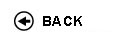Politecnico di Torino
Academic Year 2017/18
03LNMJM
Fluid mechanics
1st degree and Bachelor-level of the Bologna process in Mechanical Engineering - Torino
 Teacher Status SSD Les Ex Lab Tut Years teaching Manes Costantino A2 ICAR/01 39 18 3 0 5
 SSD CFU Activities Area context ICAR/01 6 C - Affini o integrative Attivitŕ formative affini o integrative
 Subject fundamentals The aim of the course is to give the basic knowledge of fluid properties of compressible and incompressible fluids and their behavior. The elementary tools to solve some fluid problems are given: computation of forces both in static and dynamic conditions and the analysis of fluid motion through pipelines. Expected learning outcomes Upon completion of this course, students should be able to: • Articulate the properties that distinguish fluids from other forms of matter, and the broad range of engineering applications which involve fluid mechanics. • Apply the concepts of vector fields (velocity, force acceleration), scalar fields (pressure, density, temperature), and vector differential and integral calculus to engineering analysis of fluids systems, and to the interpretation of flow physics through the conservation laws. • Properly apply Newton's second law to analysis and design involving fluids at rest using integral and differential calculus, including pressure variation, forces and moments on plane surfaces, and buoyancy. • Properly apply systems and control volume methods based on mass, momentum, and energy conservation, as appropriate, to the analysis and design of engineering fluids systems. • Correctly interpret and apply the various differential forms of the conservation laws, particularly Newton's law and its various approximate forms, to engineering analysis and design. • Properly apply mass, momentum, and energy conservation to steady internal (pipe) flows, correctly interpret and apply laminar and turbulent flow models, and estimate head loss and power requirements in piping systems. • Develop mathematical models through justifiable approximations, correctly interpret and apply the "inviscid" approximation and the "Bernoulli" relationships to analysis of fluid systems, and estimate levels of approximation in engineering models. • Apply empirical and theoretical models (if time permits, also integral methods), to the analysis of boundary layer flows, and to drag on bodies. Prerequisites / Assumed knowledge Knowledge of calculus (differential equations, integrals) and the concepts of physics related to mechanics. Contents This course is an introduction to fluid mechanics, and emphasizes fundamental concepts and problem-solving techniques. Topics to be covered include fluid properties, fluid statics, fluid kinematics, control volume analysis, internal flows (pipe flows), differential analysis (i.e. Navier Stokes equations, including approximations such as creeping flow, potential flow, and boundary layers), and external flows (lift and drag). Introduction to turbulent flows and the Reynolds equations. Students are also expected to be proficient in applying mathematics (e.g., integration, differentiation, and differential equations), statics and dynamics (e.g., free body diagrams). Delivery modes The course is divided in lectures and tutorials. Tutorials are targeted to deepen the understanding of the concepts that are addressed in the lectures. A quick visit to the laboratory is also scheduled. Texts, readings, handouts and other learning resources Fluid Mechanics: Fundamentals and Applications. Second Edition in SI Units. Y.A. Çengel and J. M. Cimbala, McGraw-Hill, New York, 2010. Assessment and grading criteria The exam is WRITTEN ONLY and includes numerical problems as well as theoretical questions (this includes derivations) that the students have to address in 2 HOURS. The students are allowed to bring only a pen and a calculator (lengthy empirical formulae will be always provided in the exam script). The exam is passed provided that a minimum score of 18/30 is achieved. Programma definitivo per l'A.A.2017/18© Politecnico di Torino
Corso Duca degli Abruzzi, 24 - 10129 Torino, ITALY